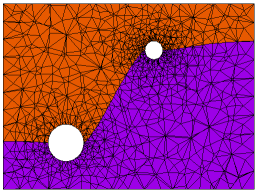﻿ Sample Problems > Usage > 3D_domains > twoz_import

# twoz_import

Navigation:  Sample Problems > Usage > 3D_domains >

# twoz_import{ TWOZ_IMPORT.PDE

This problem constructs two non-coplanar spheres inside a box using an extrusion

surface generated by TWOZ_EXPORT.PDE, which must be run before this problem.

The domain consists of three layers.

layer 1 is the space below the spheres

layer 2 contains the sphere bodies, and is of zero thickness outside the spheres

layer 3 is the space above the spheres

The sphere interiors are Void, and are thus excluded from analysis. You could just as

well fill them with material if you wanted to model the insides.

The bounding surfaces of layer 2 are specified as a default surface read from a

TRANSFER, over-ridden by regional expressions within  the (X,Y) extent of each sphere.

Click "Controls->Domain Review" to watch the mesh construction process.

See TWOZ_DIRECT.PDE and TWOZ_PLANAR.PDE for other methods of treating spheres

with centers on differing Z coordinates.

 }   title 'Two Spheres in 3D'   coordinates    cartesian3   variables    u   definitions   { dielectric constant of box filler (vacuum?) }    K = 1        box = 1 { bounding box size }     { read sphere specs from file, to guarantee      that they are the same as those in surfgen }    #include "sphere_spec.inc"     { sphere shape functions }    sphere1_shape = SPHERE ((x1,y1,0),R1)    sphere2_shape = SPHERE ((x2,y2,0),R2)   { read dividing surface generated by surfgen script }   TRANSFER("twoz_export_output/two_sphere.xfr",zbottom)    ztop = zbottom   equations    U: div(K*grad(u))  = 0   extrusion   surface "box_bottom"  z=-box   surface "sphere_bottoms"  z = zbottom   surface "sphere_tops"  z = ztop   surface "box_top" z=box   boundaries   {insulating boundaries top and bottom }        surface "box_bottom" natural(u) = 0   surface "box_top" natural(u) = 0Region 1 { The bounding box }

start(-box,-box) line to (box,-box) to (box,box) to (-box,box) to close

limited region 2 { sphere 1 }

mesh_spacing = R1/5         { force a dense mesh on the sphere }

zbottom = Z1-sphere1_shape { shape of surface 2 in sphere 1}

ztop = Z1+sphere1_shape     { shape of surface 3 in sphere 1}

layer 2 void

surface 2 value(u)=V1       { specify sphere1 voltage on top and bottom }

surface 3 value(u)=V1

start  (x1+R1,y1)

arc(center=x1,y1) angle=360

limited region 3 { sphere 2 }

mesh_spacing = R2/5         { force a dense mesh on the sphere }

zbottom = Z2-sphere2_shape { shape of surface 2 in sphere 2}

ztop = Z2+sphere2_shape     { shape of surface 3 in sphere 2}

layer 2 void

surface 2 value(u)=V2       { specify sphere2 voltage on top and bottom }

surface 3 value(u)=V2

start  (x2+R2,y2)

arc(center=x2,y2) angle=360

plots

grid(x,y,z)

grid(x,z) on y=y1  paintregions as "Y-cut through lower sphere"

contour(u) on y=y1 as "Solution on Y-cut through lower sphere"

grid(x,z) on y=y2  paintregions as "Y-cut through upper sphere"

contour(u) on y=y2 as "Solution on Y-cut through upper sphere"

grid(x*sqrt(2),z) on x-y=0  paintregions as "Diagonal cut through both spheres"

contour(u) on x-y=0 as "Solution on Diagonal cut through both spheres"

end